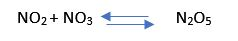# GACE Chemistry Test II (029): Practice & Study Guide Final Exam

Free Practice Test Instructions:

Choose your answer to the question and click 'Continue' to see how you did. Then click 'Next Question' to answer the next question. When you have completed the free practice test, click 'View Results' to see your results. Good luck!

#### Question 3 3. Given the following reaction, how many grams of KBr are formed if 500 g of MgBr sub 2 is reacted with 350 grams of KOH ?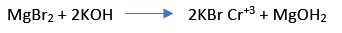#### Question 5 5. Which compound is the conjugate acid in the following reaction ?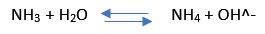#### Question 6 6. Given the redox reaction shown in the image, how many electrons must be added to its reduction half reaction in order to balance the charge ?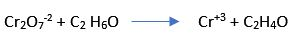#### Question 9 9. The following table lists reaction rates with the corresponding reactant concentrations for a synthesis reaction with a single reactant. What is the rate constant for this reaction ?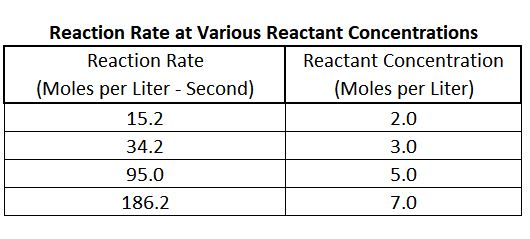#### Question 13 13. The K sub eq value for the following reaction at 25 degrees Celsius equals 2.9 × 10^-11. When the reaction is at equilibrium, what is the concentration of NO sub 3 if the concentration of N sub 2 O sub 5 is 1 × 10^-5 M ?